3D Geometry Primer: Chapter 1 - Issue 07 - From Vectors To Points by (25 September 2000) Return to The Archives
 From Vectors To PointsWell, well, goeiedag iedereen. This is already the 7th issue of this column, and the end of the 1st chapter is in sight. It's about time to dream of the 2nd one... things we could do with points, once we know them. We could build lines and planes (Ooh!), spheres and other curved things... But that's not the point ... yet. First, we still need to step from vectors to points. And that's what we're going to try this time. So, relax, and take out a sheet of paper to scribble on. Surrender yourself to the wonderful world of vectors. Do not try to fight it, because the vectors would attack you where you don't want them to...

 (I) Pull A Vector To That Point: Place VectorsHow do you step from vectors to points? Well, actually, you do the reversed thing: you step from points to vectors.Let me explain. Everybody knows how to find, in the real world, the middle of 2 different points. There're many ways to do this, and you have probably never used vectors to do this (at least in the real world). If you can't do this with a compass (remark 1), then you can always do it with a measuring rod. And the nice thing about all those methods is: it really doesn't matter where you're doing this. Is it on a sheet of paper? Is it in your garden? Is it in your car? It always gives the same result: the middle of the points.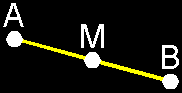How different this is, if we want to find the middle of 2 points in our virtual 3D world. We can't use that compass anymore, no measuring rod can do the job. Besides, we still can't even store those points. How do we expect then, that we can find the middle? So, we still need a way to store those points into our computer. How?Pull a vector to it! Tadaa! We already know how to store vectors and how to work with them. So, if we could represent all points by vectors, we have done the job. The idea is, pull vectors to each point. This is: each point is represented by a vector, of which the head is that point. This is a bit of a tricky sentence, so read it once more: This is: each point is represented by a vector, of which the head is that point. The point itself has become the ending point of that vector.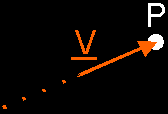So far, we always ignored the ending point of vectors, because they didn't have a real meaning. But here they do. This type of vectors, whose heads (ending points) represent points, are called place vectors. Each point has its own place vector (not really, but this is good enough for now). Remember that (for now) these are the only vectors that have a real ending point. All other vectors still have that virtual, meaningless ending point.OK, OK, we said pull a vector to it. You're probably asking the same question in your head over and over again, because you know that vectors also have starting points: "Where do we pull it from?" But you also know the answer already: the origin. All place vectors start in this point and end in their head, in *their* point. This is *the* key to connecting points to vectors. No origin is no connection.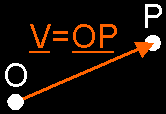Mostly, I use 2 letters to indicate a place vector. It's another way to write down bounded vectors (which place vectors are) and is already mentioned in the first issue. It consists of writing the tail and head points together and underscoring it as a whole. The tail is always an origin and the head is always point concerned. e.g. the place vector of point P is OP.This manner to write down place vectors has some benefits: 1. You immediately see of which point this place vector is. Look to the 2nd letter. 2. If you use multiple origins, you can immediately see which origin is used for this place vector. Look to the 1st letter. 3. You immediately recognize this as a place vector, because I don't use this manner for anything else. 4. You save some letters. If you don't do it this way, you have to look for another letter to write down the place vector. e.g. the place vector of P would become V. But now, we can use this letter for other purposes.Let's recapitulate all this. We connect points to vectors by choosing a privileged point: the origin. From that point we pull place vectors to each point in space. That point is the ending point of its place vector. The origin is the starting point of that vector. You store a point in your computer, by storing nothing else but it's place vector.Notice that place vectors are bound vectors! They are bound to the origin.

 (II) Place Vectors Are NOT UniqueNotice how place vectors depend on the origin (and on its ending point as well, of course). If you choose another origin, then you will find other place vectors. This is illustrated in next image. Originally, we had choosen origin O and the place vectors of points P and Q are clearly OP and OQ respective. But now, we want to change the origin and we choose point O'. Then the place vectors are O'P and O'Q, and these are definitely different vectors than the original ones.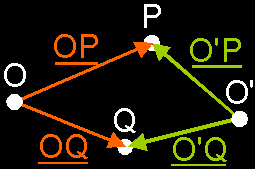This is something you always have to keep in mind: PLACE VECTORS OF POINTS DEPEND ON THE CHOSEN ORIGIN. This will influence point arithmetics.

 (III) Extension Of The Vector Base To Our Cartesian Coordinate SystemWhat A Title! You probably already know what the cartesian coordinate system is, don't you. Here, we use a righthanded orthonormal coordinate system. Well, just look at this picture: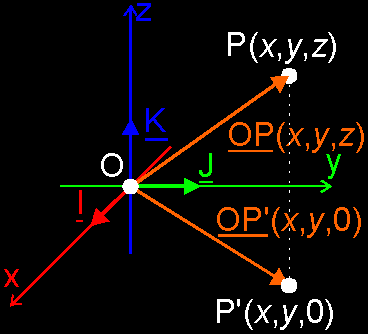The origin of our vector space is, of course, also the origin of our cartesian coordinate system.The different axes (x, y and z) fall together with the base vectors (I, J and K): x with I, y with J and z with K. Each base vector has the same direction and sense like each axis. Notice the non-italic small letters that indicate lines and axes.All base vectors have magnitude 1, so their heads fall together with the unit points on the axes. Through this, the base vectors are the place vectors of the unit points. And it's because of this that the unit points are called I(1,0,0), J(0,1,0) and K(0,0,1).But, it's up to you to realize the next thing, it's already mentioned in the picture. The coordinate values of a point are the same values as the component values of its place vector. What a sentence! I can't really explain it, you have to see it:Take a point e.g. A(1,2,3) and pull its place vector to it. What are the component values of the place vector? What linear combination of the base vectors you need, to construct that place vector? Try some other points and then, you need to make the step to a general point P(x,y,z). Do you really see that the component values of its place vector OP is (x,y,z) too? This should illustrate that when we talk about points in our numerical 3D space, we immediately have to see the vector behind the point.You definitely need to see that if you store your point in the computer, because you store the place vector instead of the point. In plain English, you actually store the coordinates of the point in a vector. In this way, the place vector and the point itself have become - in your computer - *one* object: a vector object. If it is so in your computer, you should do the same in your head: always consider a point and its place vector as one object!

 (IV) Does (Numerical) Vector Arithmetic Depends On Its Vector Base?First, one more other thing before we continue on points. Imagine that all the stuff you've just learned doesn't exist, that you don't know how to step from points to vectors...The results of all vector operations you do, does it depend on the choice you make about the base vectors, or even the origin? If you do e.g an additon, can it has more than one result, depending on the base? The answer is simply: NO. Why not?a) Can it depend on the vector base? No, because all operators, all arithmetic have been laid down and explained, before we have even introduced vector bases. So, it simply cannot have anything to do with it. It didn't existed yet at the time we learnt about vector operations. Do you remember all those little drawings?A vector base is nothing more than an instrument to translate vector stuff to numerical stuff. It's just a way to store the vectors.b) Can it depend on the origin? No, because until now, we've always used free vectors. And free vectors do not care about starting points (they simply don't have it). The so called origin is nothing more than a point where you gather all information. You can pick any point to do that. It doesn't change anything to the results.In fact, you cannot depict a free vector itself. You remember that a free vector is a set of all bound vectors with the same direction, sense and magnitude. So, what you actually do if you depict a free vector, is to take 1 representative of that set (1 bound vector) and depict that one. Thus, if you gather all information in a origin, you just take that representative of each free vector, that has its starting point in that origin.c) By Analogy With Colors... You can use several methods to describe a color. You can tell its RGB components, or use the CMYK method, and there many other ways. But none of them influence the way how 2 different colors will mix. If you know the "component values" of the original colors, you will be able to calculate, to predict, the values of that mix. And those values will be correct: each model will end up with different but correct values of the same result. The result is not influenced by the choice of the model to do the calculation. It's in that way you have to think about numerical vector arithmetic.

 (V) The Role Of The Origin In Point ArithmeticThe origin is only a privileged point once you've chosen one. You can choose any point as origin, but once you've made your choice, all other points and all place vectors have to bow to the great origin. That's something we don't have in the real world.We have to keep in mind that THE REAL WORLD HAS NO ORIGIN. If we want to create a good model of that real world, we have to make sure that the reality is not influenced by that model. The meaning of "the middle of 2 points" may not change because we use a model. It has to behold its real meaning.Therefore, the perfect model wouldn't have an origin either. But, unfortunately, we really need that origin to store those points. So, we have to find a way of point arithmetic that is not influenced by it (although it is a privileged point). The special character of that origin makes it quite difficult.How shall we solve that problem? We'll see that next week. But now, I'll say goodbye. Where the wild things are...

 Remarks(1) I was very suprised when I saw that compass is the translation of what we call in Dutch: een passer. You know, that thingy with 2 legs you can use to draw circles. At least this is what my dictionary told me. I always thought that a compass was just that thing to find the North and the South. How do you guys know the difference?